• 最小二乘参数估计的递推算法及其C语言实现 精品.pdf
2021-05-26 00:36:45

2009年4月 焦作大学学报 №．2

第2期 JoURNALOFJIAOZUOUNIVERSITY Apr．2009

最小二乘参数估计的递推算法及其C语言实现

胡 沙

(河南理工大学电气工程与自动化学院，河南焦作454003)

摘要：最小二乘参数估计的递推算法是系统参数辨识中最基本、最成熟的方法。文章首先介绍了最小

二乘法的递推算法原理和本识别系统的框架流程图，然后针对文章的算法分别阐述了服从N(0，1)正

态分布自相关随机噪声v(k)的产生方法。文章着重介绍了利用c语言编程对一个简单系统的参数

辨识实现最小二乘参数估计的递推算法，详细说明了本系统各个环节的C语言实现，并通过matlab

仿真对数据进行了详细的分析。从仿真实验结果可以看出，试验数据符合试验要求，系统的参数辨识

实现最小二乘参数估计的递推算法的效果令人满意。

关键词：最小二乘参数估计的递推算法iC语言；matlab

中图分类号：TP312文献标识码：A 文章编号：1008—7257(2009)02—0082—03

模型结构。

1．最小二乘参数估计的递推算法原理

z

z(k)+alz(k一1)+a2z(k一2)=blU(k一1)+b2

最小二乘参数估计的递推算法是系统参数辨识中 (k一2) (5)

最基本、最成熟的方法。递推算法的基本思想可以概括

成：新估计值0(k)=老的估计值e(k一1)+修正项。(0)=106I，0(0)=0．001

0(k)=O(k一1)+K(k)[z(k)一“。(k)o(k—1)]

(1)3．服从N(0，1)正态分布自相关随机噪声

K(k)=P(k一1)h(k)[h1(k)

v(k)的产生方法

(2)

P(k—1)h(k)+志]“ 3．1(O。1)均匀分布随机数的产生

P(k)=[I—K(k)h’(k)]P(k一1)(3) 本文用了乘同余法产生(0，1)均匀分布随机数，L

为了保证对称性，把(3)改为 {}表示取小数部分，{专，l是伪随机序列。

P(k)；P(k一1)一K(k)KT(k) 玉=L(越¨) (6)

(4)

[h7(k)P(k—1)h(k)+÷]一 、’7(7)

』～ 岛=罟

bo—M

A s

s3(mod8)或A5(mod8)且A不能太小，初值

2．本文要辨识的系统

】【0取正奇数，M为2的方幂，即M=2。，k>2。本文取A

系统如图1。

=179，M=2加，xo=1l。

3．2正态分布随机数的产生

更多相关内容
• 递推最小二乘实现参数估计，对不确定的系统有良好的参数估计效果。
• 遗忘因子递推最小二乘参数估计，用于识别系统，MATLAB程序 遗忘因子递推最小二乘参数估计，用于识别系统，MATLAB程序
• 最小二乘参数估计的递推算法及其C语言实现.pdf
• 递推最小二乘算法递推最小二乘算法递推最小二乘算法递推最小二乘算法递推最小二乘算法递推最小二乘算法
• 在此代码中，我们考虑了MIMO OFDM系统的最小平方误差信道估计。 用户可以访问MIMO OFDM系统的设计参数和信道状态信息。 L抽头瑞利衰落信道被视为在任何一对发射天线和接收天线之间。 将仿真结果得到的LSE信道的均方...
• 本资源为利用递推最小二乘算法对永磁同步电机的所有四个参数（定子电阻，转子磁链，d轴和q轴电感进行在线估计的仿真模型和有逆变器死区补偿的参数辨识模型
• 梳理经典最小二乘算法，及加权最小二乘和总体最小二乘，重点关注不同算法间的逻辑联系

本文主要讲标准最小二乘方法及其常见的变形：加权最小二乘和总体最小二乘算法，关注不同方法之间的逻辑。

# 一、最小二乘估计（Least Squares estimation，LS）

最小二乘估计方法是一种不需要先验知识的常见参数估计方法。

假设信号模型为：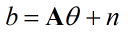在雷达信号中，A为方向矢量，b为阵列接收信号，θ为原始目标信号，n为噪声。更一般的A为观测的系数矩阵，b为观测向量。

A常见有三种情况

1.当A为未知参数等于方程数，则上述方程为适定方程，存在唯一解

2.当A为未知参数小于方程数（行数多于列数），则上述方程为超定方程

3.当A为未知参数大于方程数（行数小于列数），则上述方程为欠收方程

一般雷达系统中最常见的为超定方程，为接收阵列数大于目标数。

最小二乘估计参数的代价函数，该准则为使误差的平方和最小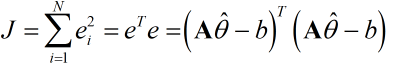代价函数J对未知变量θ求导，令求导结果为0，得到最小二乘的估计为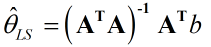如果矩阵A是秩亏时，则称该参数是不可辨识的。

但是当误差向量的各个分量具有不同方差，或者各个分量是不相关的，则标准的最小二乘估计不再是最优的，引出需要寻找更一般的方法。

Gauss-Markov定理

该定理表示超定方程下，如果误差的向量的均值为零矩阵，方差矩阵为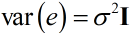则该回归方程的最佳线性无偏估计的标准的最小二乘估计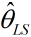。因此当误差向量不满足该定理下的假设时，需要变换到该假设下，进而能得到最佳的估计值。

# 二、加权最小二乘（Weighted Least Squares estimation，WLS）

当误差向量的方差为为以下的构造形式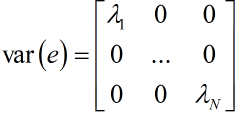对角线上方差值各不相同，但是对角线外元素为0，不满足Gauss-Markov假设，因此构造一个加权的代价函数，加权误差平方和，来作为优化的目标函数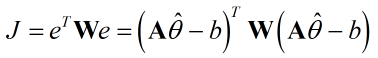再令代价函数最小，对位置参数θ的估计值求导，可得加权最小二乘估计值为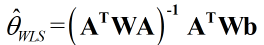但是问题是，如何确定加权矩阵。根据误差向量的特定形式且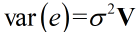矩阵V满足正定矩阵的结构，现代信号处理第三版_张贤达在p43式(2.6.8)有解释新的观测模型的误差向量的方差矩阵为单位矩阵和一常数的乘积，因此满足Gauss-Markov的假设。所以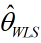加权最小二乘估计的权值由误差的方差确定对加权最小二乘估计的物理解释为，由于噪声的协方差的对角元素值各不相同，根据各自的数值赋予该接收信号权值。如某个元素噪声过大，则与其对应的阵列接收信号向量则受噪声污染更严重，赋予其更小的权值，更愿意相信被噪声污染不严重的接收信号向量。

# 三、广义最小二乘（Generalized Least Squares estimation）

LS估计和WLS估计都是GLS估计的一种特殊形式，当噪声方差矩阵主对角上各元素不相等，同时非对角线上还有非零数值时，即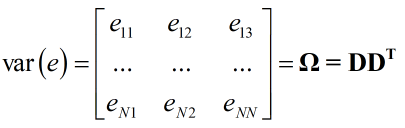LS和WLS的估计值都非最优的，根据噪声方差矩阵的形式构造一个新的模型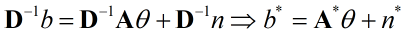接着对构造的新模型做LS估计得到最优估计结果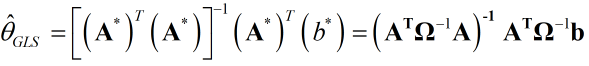# 四、总体最小二乘（Total Least Squares estimation）

实际情况中，除了噪声的扰动导致接收信号有误差之外，系数矩阵A也会含有误差，此时应该同时考虑到接收信号b和系数矩    阵A二者的误差或者扰动，即新的信号模型修改为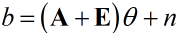TLS估计的思想是使来自A和b的噪声扰动影响最小，根据信号模型，构造新的等式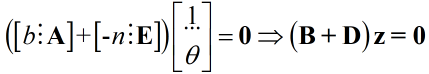令扰动矩阵D的Frobenious范数最小，其中扰动矩阵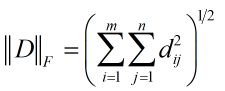求该优化方程的方法用到了奇异值分解做最佳逼近；大致过程为求增广矩阵B的奇异值分解，确定B的秩，利用奇异值分解的结果构造逼近矩阵B的估计。接着构造一个逼近矩阵的某些向量构造相关矩阵S，TLS的估计值为S矩阵的逆的归一化数值。具体过程在现代信号处理p133中。有机会详细说一下该过程的核心思想。

# 五、自适应最小二乘（Recursive Least Squares estimation）

这里是最小二乘的自适应实现，考虑了一种指数加权的最小二乘方法，代价函数为指数加权的误差平方和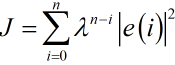有机会详细讲迭代自适应方法及一些典型的应用。

其他：

矩阵求逆引理

矩阵求逆引理(matrix inversion lemma)_家家的专栏-CSDN博客_矩阵反转引理MATLAB

“Line Fitting with Online Recursive Least Squares Estimation” 是MATLAB给出的一个迭代最小二乘参数估计的例子。

贴出来部分结果，感兴趣可以自行查看matlab相关文档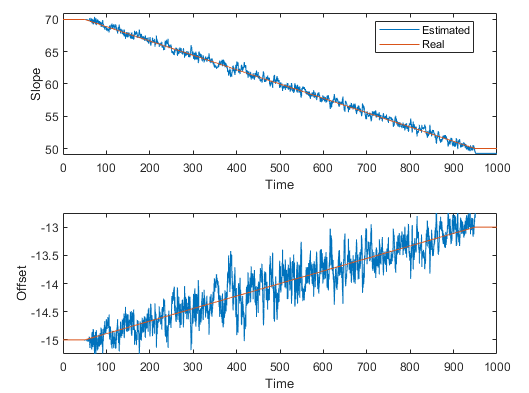用来估计的offset和slope参数来做直线拟合。

展开全文矩阵
• 递归最小二乘算法完成状态观测，用于求解状态，进行状态估计
• 系统辨识最小二乘参数估计matlab.doc 《系统辩识与自适应控制》最小二乘参数估计摘要：最小二乘的一次性完成辨识算法(也称批处理算法)，他的特点是直接利用已经获得的所有(一批)观测数据进行运算处理。这种算法在...系统辨识最小二乘参数估计matlab.doc

《系统辩识与自适应控制》最小二乘参数估计摘要：最小二乘的一次性完成辨识算法(也称批处理算法)，他的特点是直接利用已经获得的所有(一批)观测数据进行运算处理。这种算法在使用时，占用内存大，离线辨识，观测被辨识对象获得的新数据往往是逐次补充到观测数据集合中去的。在应用一次完成算法时，如果要求在每次新增观测数据后，接着就估计出系统模型的参数，则需要每次新增数据后要重新求解矩阵方程。ZlTlT1最小二乘辩识方法在系统辩识领域中先应用上已相当普及，方法上相当完善，可以有效的用于系统的状态估计，参数估计以及自适应控制及其他方面。关键词：最小二乘(Least-squares)，系统辨识(SystemIdentification)目录：1.目的.22.设备.23引言.23.1课题背景24数学模型的结构辨识.35程序45.1M序列子函数.45.2主程序56实验结果：.77参考文献：.71.目的1.1掌握系统辨识的理论、方法及应用1.2熟练Matlab下最小二乘法编程1.3掌握M序列产生方法2.设备PC机1台(含Matlab软件)3引言3.1课题背景最小二乘理论是有高斯(K.F.Gauss)在1795年提出：“未知量的最大可能值是这样一个数值，它使各次实际观测值和计算值之间的差值的平方乘以度量其精度的数值以后的和最小。”这就是最小二乘法的最早思想。最小二乘辨识方法提供一个估算方法，使之能得到一个在最小方差意义上与实验数据《系统辩识与自适应控制》最好拟合的数学模型。递推最小二乘法是在最小二乘法得到的观测数据的基础上，用新引入的数据对上一次估计的结果进行修正递推出下一个参数估计值，直到估计值达到满意的精确度为止。4数学模型的结构辨识4.1根据汉格尔矩阵估计模型的阶次设一个可观可控的SISO过程的脉冲响应序列为{个g(1),g(2),……g(L)},可以通过汉格尔(Hankel)矩阵的秩来确定系统的阶次。令Hankel阵为：，其中决定阵地维)2()1()(2)1()()(),(lkgkglkgllHl),(klH数，k可在1至间任意选择。则有。2LnlHran,,,0如果(过程的真实阶次)，那么Hankel阵的秩等于。因此可以利用Hankel0nl0阵的奇异性来确定系统的阶次。0n4.2根据残差平方和估计模型的阶次SISO过程的差分方程模型的输出残差为，数据长度L，为阶时的数据矩阵，)(~kznHˆ为阶时的参数的估计量，为模型阶次估计值，为真实阶次，则残差平方和函数：nˆnˆ0nJ)(~1)ˆ()ˆ(1~)ˆ(2ˆ00kzLzHzLzJnknTnnnT残差平方和有这样的性质：当L足够大时，随着增加先是显著地下降，当>ˆJnˆ时，值显著下降的现象就终止。这就是损失函数法来定阶的原理。0n)ˆ(J图1-1损失函数法原理。。。。。。。。。0nnˆ《系统辩识与自适应控制》4.3根据AIC准则估计模型的阶次)ˆ()ˆ(1ˆ21MLnTMLnvLHzzAICv4log)2具体的定阶用法是：对不同阶次首先用极大似然法估计参数，然后计算似然函数值及值，找到使的作为。)ˆ(nImin)ˆ(Iˆ05程序%待辨识系统z(k)=0.1*z(k-1)-0.5*z(k-2)+0.6*z(k-3)+u(k-1)+0.5*u(k-2)-0.2*u(k-3)+v(k)/800%clcclear%清理工作间变量L=300;%M序列的周期x1=1;x2=1;x3=1;x4=0;x5=1;x6=0;%四个移位积存器的输出初始值fork=1:L;%开始循环，长度为Lu(k)=xor(x3,x4);%第一个移位积存器的输入是第3个与第4个移位积存器的输出的“或”x6=x5;x5=x4;x4=x3;x3=x2;x2=x1;x1=u(k);end%大循环结束，产生输入信号uplot(u)%绘图M序列v=randn(300,1);%随机误

展开全文• 借助切换函数简化系统模型, 通过损失数据模型估计系统损失的输出数据, 进而利用系统所有输入和输出数据, 提出相应双率系统递推最小二乘算法. 与多项式转换方法相比, 该方法能够直接辨识出系统参数. 仿真结果验证了...
• 利用最小二乘递推算法进行辨识

## 1.1 问题描述## 1.2 方法思路

模型类选CAR模型##1.3 实验结果## 1.4 实验代码（Matlab）

main.m

%%%%采用递推最小二乘法对四个真值[a1 a2 b1 b2]=[-1.5 0.7 1 0.5]进行估计
%%%第一步：选用的模型类为CAR模型，由已给的条件可知模型阶数为2
%产生M序列做为输入，周期为1023，幅值为1
u=F2(1023,1);%%利用作业2中代码封装成的函数产生周期为1023的M序列
%产生均值为0，方差为1的高斯白噪声
V=F1(0.1,0,550);%%利用作业1中代码封装成的函数产生白噪声
%四个参数估计值
Y=zeros(4,501);
%任意给定Y初值
Y(:,1:2)=[1 2 -1 3;0.8 -2 1 3]';
%给定P（0）满足课本式5-2-19，使P（0）尽量大
P=5000*eye(4);
%给定增益矩阵初值
K=[1 1 1 1]';
%任意给定Z初值
Z(1:2)=[0 1];
%根据课本式5-1-1计算z(k)
for k=3:502
Z(k)=1.5*Z(k-1)-0.7*Z(k-2)+U(k-1)+0.5*U(k-2)+V(k-2);
end
%%%第二步：由课本式5-2-16，另加权因子为1，得到普通最小二乘递推算法RLS，其递推公式如下：
for k=3:502   %递推500次
%%由课本式5-1-1可以得到h(k)的表达式，模型阶数为2，na=nb=2
h=[-Z(k-1) -Z(k-2) U(k-1) U(k-2)]';%此时的h为第k次的值
%%由课本式5-2-16可得RLS表达式如下：
K=P*h*inv(h'*P*h+1);%此时的P为第k-1次的值,h为第k次的值
Y(:,k)=Y(:,k-1)+K*(Z(k)-h'*Y(:,k-1));%此时的K为第k次的值,h为第k次的值
P=(eye(4)-K*h')*P;%此时的P为第k次的值
end
%%%第三步：画图，并比较递推500次后的估计值与真值
figure
t=[1:1:502];
%%画出a1的估计值
plot(t,Y(1,:),'r')
e1=-1.5-Y(1,502);%a1与真值的误差
text(502,Y(1,502),'a1');
hold on
%%画出a2的估计值
plot(t,Y(2,:),'g')
e2=0.7-Y(2,502);%a2与真值的误差
text(502,Y(2,502),'a2');
hold on
%%画出b1的估计值
plot(t,Y(3,:),'b')
e3=1-Y(3,502);%b1与真值的误差
text(502,Y(3,502),'b1');
hold on
%%画出b2的估计值
plot(t,Y(4,:),'m')
e4=0.5-Y(4,502);%b1与真值的误差
text(502,Y(4,502),'b2');
title('递推500次后的参数估计值的跟踪曲线')
legend('a1','a2','b1','b2')


F1.m

function Y3=F1(v,m,n)
%利用乘同余法和变换抽样法产生均值为m，方差为v的正态分布的白噪声
%公式：Xi=A*Xi*mod(M)
M1=2^11;%为2的方幂
M2=2^17;%为2的方幂
A1=119;%%不能太小
A2=279;%%不能太小
x1=1;%伪随机序列1的初值
x2=11;%伪随机序列2的初值
X1=x1;
X2=x2;
Y1=[];%伪随机序列1
Y2=[];%伪随机序列1
% n=700; %伪随机序列长度
%%%%%采用乘同余法产生伪随机序列%%%%%
for i=1:n
%产生伪随机序列1
X1=mod(A1*X1,M1);
Y1=[Y1 X1/M1];
%产生伪随机序列2
X2=mod(A2*X2,M2);
Y2=[Y2 X2/M2];
end
%正态分布的白噪声
Y3=m+v*sqrt(-2*log(Y1)).*cos(2*pi*Y2);%正态分布的白噪声
figure
plot(Y3,'b');
title('正态分布的白噪声');
end


F2.m

function X=F2(T,a)
%%%采用伪随机信号发生器生成周期为1023的M序列
y=[1 1 0 1 1 0 1 0 1 0];%%%初始化10级移位寄存器，保证其初值不全为0
for i = 1:1:T
Y(i)=y(10);%%%第n级的输出即是M序列的两种状态
M(i)=mod(y(10)+y(7),2);%第n级与第N1级模二加结果，此时分别为10和7
%%%%移位寄存器工作原理%%%
y(10)=y(9);
y(9)=y(8);
y(8)=y(7);
y(7)=y(6);
y(6)=y(5);
y(5)=y(4);
y(4)=y(3);
y(3)=y(2);
y(2)=y(1);
y(1)= M(i);
end
for i=1:1:T
if Y(i)==1
X(i)=a;
else
X(i)=-a;
end
end
stairs(X,'b'); %画出伪随机信号
title('周期为1023、幅值为1的伪随机信号');
ylim([-1.2 1.2])
end

展开全文matlab
• 文章目录写在前面收获参考文献 写在前面 写了好几个关于梯度下降的pyhton脚本，里面都提到了最小二乘法，但是我对最小二乘法...最经典的回归模型参数估计算法最小二乘 Ordinary Least Square(OLS) 普通最小二乘 ...回归 机器学习
• 针对有色噪声干扰的输出误差滑动平均系统,将辅助模型与递推增广最小二乘算法相结合:用辅助模型的 输出代替辨识模型信息向量中的未知真实输出项,用估计残差代替信息向量中的不可测噪声项,从而提出了基于辅助模型的...
• 文章目录Reference最小二乘估计加权最小二乘估计 Reference Matrix Differentiation 加权最小二乘法与局部加权线性回归 卡尔曼滤波与组合导航原理(1-2讲) 最小二乘估计 Z=HX+VZ = HX + VZ=HX+V where, Z is the ...
• 此压缩包里已给出白噪声数据，左列为输出数据，右列为输入数据。此算法最小二乘参数估计的递推算法
• 在工程物理、 化学工程、 生物医学、 统计学、 经济学、 信号处理、 自动化、测绘学等领域中， 许多问题都可归结为求解矩阵方程 Ax=b的问题，其中最常见的是线性参数估计问题，而最小二乘法是最常用的线性参数估计...
• 递推最小二乘估计Matlab仿真递推最小二乘估计理论仿真背景Matlab程序仿真动图 递推最小二乘估计理论 递推最小二乘是卡尔曼滤波的重要基础，关于最小二乘理论可以参见之前的文章（ 最小二乘估计（Least squares ...matlab
• 基于最小二乘估计方法，实现线性回归方程中回归参数估计。并且和statsmodels中的方法进行对比。 1.线性模型和最小二乘方法 线性模型是指预测值是特征（feature）的线性组合（liner combination），数学表达式如下...python 机器学习
• 最小二乘估计概念 古典最小二乘估计 tic clc; clear; %首先假定量测量值如下 mul=[100,200,300]; sigma=[4,0,0;0,4,0;0,0,16]; data=mvnrnd(mul,sigma,100); %假定量测量Z是按照100个x，y,z交替排列 Z=zeros(300,1...
• 提出了基于复数U-D分解的复参数最小二乘估计方法。在传统的加权遗忘因子法的递推算法中，方差矩阵P（k）由于衰减很快而极易失去正定性。为了保证参数估计的收敛性，利用复数U-D分解，将方差矩阵P（k）进行U-D分解，...
• 最小二乘估计矩阵形式的推导 最近写文章有用到一些算法，自己推一下，顺便mark下来。 这么久没上csdn居然都能写Tex了(666) 考虑一般线性回归模型(OLR) 考虑只含有一个指标的一般线性回归模型(ordinary linear ...
• 给出了增删数据场合多元线性回归模型参数最小二乘估计的两种算法―递推算法和Givens变换法,二者都利用已有的结果,减少计算量.递推算法给出预测误差的关系式,可直接比较预测误差大小;Givens变换法不能直接导出预测...
• 本文重点研究维纳非线性系统的... 为了解决信息向量中具有不可测变量的维纳非线性系统的辨识问题，提出了一种基于最小二乘的迭代算法，将信息向量中的不可测变量替换为其对应的迭代估计。 仿真结果表明该算法是有效的。研究论文
• 系统辨识最小二乘参数估计matlab.doc 《系统辩识与自适应控制》最小二乘参数估计摘要：最小二乘的一次性完成辨识算法(也称批处理算法)，他的特点是直接利用已经获得的所有(一批)观测数据进行运算处理。这种算法在......